Alternating Current

Alternating Current and the generation of a sinewave

Introduction

The household electrical supply and the signal from a radio transmitter are similar. Both are AC (alternating current) signals, but they differ in frequency. We will first consider how a simple generator / dynamo / alternator works to produce electricity  and then consider typical radio frequency signals.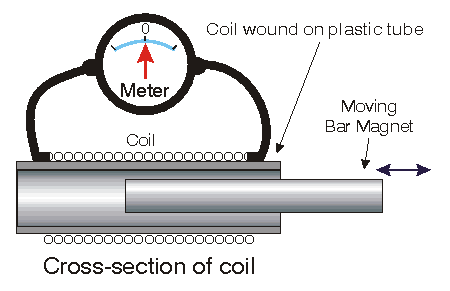Consider a coil of fine insulated wire wound on a plastic or cardboard tube. Connect the ends of the coil to a DC milliammeter or galvanometer (for demonstration purposes!). A center-zero meter is preferred for this demonstration.

Now take a bar magnet and thrust the magnet into the coil. The meter will deflect.

Now withdraw the magnet. The meter deflects the other way.

As we insert and withdraw the magnet, the meter deflects from one side to the other.

With the magnet stationary, the meter reads zero.

What's happening?

We see that when the magnet is moving, a current is induced in the coil. The moving magnetic field, from the moving magnet, cuts the conductors forming the coil.

We can, by this simple experiment, deduce that the amplitude of the current depends on several things:

the strength of the magnet,

the rate of cutting of the conductors (i.e. the speed of movement of the magnet) and

the number of conductors (i.e. the number of turns on the coil).

We are converting mechanical energy (the movement of the magnet) into electrical energy. We have demonstrated Faraday's law of induction.

Other considerations                                                                                        To top of page

This conversion of mechanical energy (the movement of the magnet) into electrical energy, has other important aspects too.

The magnet could be stationary and the coil could move - we will consider this case again below.

This principle is the basis of generators, meters, loudspeakers, relays, and lots of other things too.

Consider the reverse operation too: A current could be fed to the coil. This would force the magnet to do the moving.

The magnet need not be a permanent magnet - it could be another coil, wound over the top. We could feed an AC signal to one coil, the rising and falling magnetic field from it sweeps across the other coil, generating an induced signal in the second coil (the secondary winding). The two coils are insulated from each other, the coupling is by the sweeping magnetic field. Nothing needs to make any physical movement. So we have a transformer

Frequency

Placing the magnet into the coil and then removing back to the start point is one cycle. If we could take the magnet in and out of the coil every second, the polarity of the induced voltage would go through one cycle in one second. One cycle per second is one hertz (Hz).  So when you see 'hertz' or 'Hz' just think of 'cycles per second'.

The alternating voltage provided to your house from the power grid repeats polarity 50 times a second and reaches 230 volts r.m.s. (the "rms" will be treated below). It is 230V 50 Hz.

The AC supply causes the electrons in the conductors in your house to first move one way and then the other. They are probably the same electrons that were there when the wiring was first installed. They just seesaw back and forth.

The device which creates your household power is called a generator. It works by creating relative motion between a conductor and a magnetic field.

The Generator                                                                                                    To top of page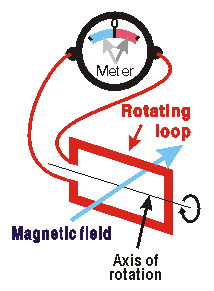Consider a magnetic field that is constant in its intensity. In this field is a rotating coil. For simplicity, this diagram on the left shows it as a single-turn loop. The ends of the coil are brought out to a meter - the same type of meter as before. In practice, slip-rings would be used to prevent the wires tangling with rotation - but this complication has not been shown here.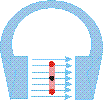Please note that this meter has red and blue scale sections to show the change in polarity of the current as the coil rotates.

The coil is rotated in the constant magnetic field by mechanical means (in practice by water power or by diesel power etc.). Of course the design of a real generator is quite different - we are looking at the simple principles.

We are considering the current induced in each of the longer elements of the loop only - so please ignore any complications brought on by the end parts of the loop!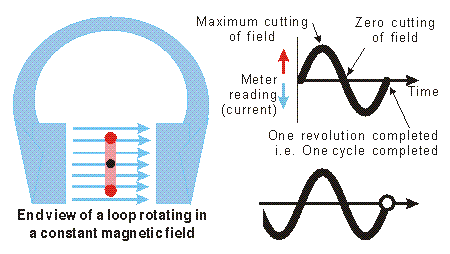When the plane of the loop is vertical between the jaws of this magnet, the loop elements are moving parallel with the direction of the magnetic field. The loop is not cutting any lines of force. So the meter will read zero.

When the plane of the loop is horizontal, the loop elements will be moving vertical in the jaws of this magnet, a maximum cutting of the magnetic field. So the meter will read maximum current.

As the loop rotates, the meter will read a current first in one direction, pass through zero, and then show a current in the other direction, then back to zero - one full cycle. It is shown here by the red and blue readings on the meter - a variation of our first example when we used a meter with a bar magnet.

Consider the angle made by the the plane of the loop with any vertical line on the diagram. This angle is significant. When the plane of the loop is vertical in the diagram, the plane of the loop is at zero degrees with a vertical line. When the plane of the loop is horizontal, this angle is 90 degrees.

The output current indicated on the meter follows this angle change - a sinewave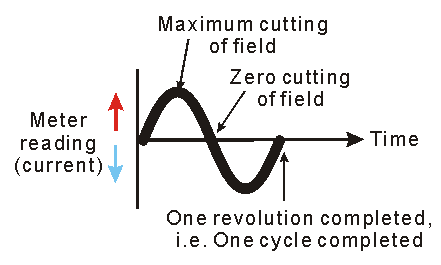At 30 degrees, the current will be sin30 = 0.5 of the maximum, at 45 degrees, the current will be sin45 = 0.707 of the maximum, at 60 degrees, 0.866, and so on.

The frequency of the wave is related to the revolutions, one revolution produces one cycle. One cycle in one second is a 1 Hz signal.

The 50 Hz mains supply can be produced by mechanical means, and examples are in every powerhouse supplying the national grid. Fifty cycles in one second (50 Hz) is 3000 revolutions in each minute. Multi-polar machines are used in practice with a slower rotation speed.

It is difficult to produce radio frequencies by mechanical means so these are generated by electronic means. For example 7 MHz represents 7 million revolutions in one second. You can work out how many in one minute!

In practice, the hertz, Hz; the kilohertz, kHz; the megahertz, MHz; and the gigahertz, GHz; are used in radio work.

The time for one period

The time taken for one cycle, or one period, is the reciprocal of the frequency.

For example, a frequency of 50 cycles in one second, (i.e. 50 Hz), means that the time for one period is one-fiftieth of one second.

The time for one period of a 7 MHz signal is one-seven-millionth of one second, or one-seventh of one microsecond.

Harmonics

It is difficult to produce a pure sinewave. Signal impurities known as "harmonics" are exact multiples of the original fundamental signal. The harmonics can be odd-multiples or even-multiples of the fundamental signal, or both.

The presence of harmonics has the effect of altering the resulting wave-shape from being sinusoidal to being some other distorted waveform. The level of harmonic content can be very small and the wave-shape changes are often not detectable without the use of test equipment to determine the relative level of the harmonic frequency components.

Harmonics bring interference problems in radio operating too.

Question 1: For a 230V 50 Hz mains supply, what is the Peak Voltage?

Question 2: How many times in each cycle is this Peak Voltage reached?

____________________________________________